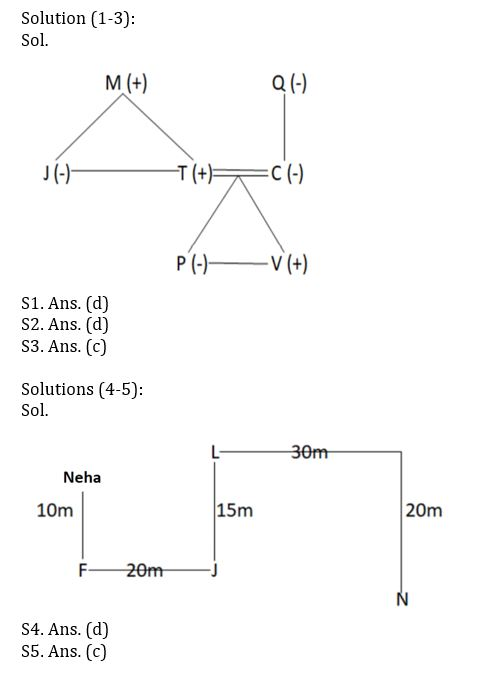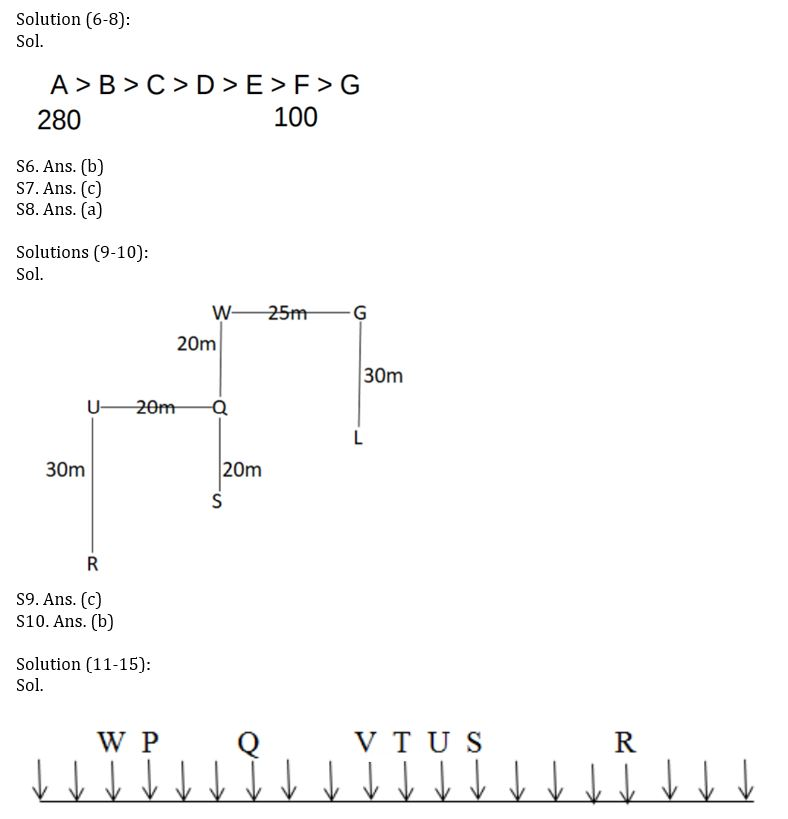Latest Banking jobs   »   Reasoning Ability Quiz For FCI Phase...

# Reasoning Ability Quiz For FCI Phase I 2023- 15th January

Directions (1-3): Study the data carefully and answer the questions accordingly.
There are seven members in a family of three generations. Q is a mother-in-law. J is the aunt of P who is the granddaughter of M. V is the grandson of Q, who is not married to M. M is the father of J and T. C is the mother of P and V.

Q1. Find the correct statement from the following.
(a) T is the son of Q
(b) C is the daughter-in-law of Q
(c) J is the sister of C
(d) M is the father-in-law of C
(e) None is correct

Q2. How V is related to T?
(a) Nephew
(b) Bother
(c) Uncle
(d) Son
(e) None of these

Q3. Who among the following is a couple?
(a) Q, T
(b) C, M
(c) T, C
(d) V, J
(e) None of these

Directions (4-5): Study the data carefully and answer the questions accordingly.
Neha starts walking towards south and covers a distance of 10m to reach point F. Now, she took a left turn and reached point J after a distance of 20m. From here, she moved towards north and reached point L after a distance of 15m. Finally, she took two consecutive right turns and walked 30m and 20m respectively to reach point N.

Q4. Which of the following is true?
(a) Point J is in south-west of point N.
(b) Point N is in west of point F.
(c) The shortest distance between the points L and N is 10√11
(d) The shortest distance between the points J and N is 5√37
(e) None is true

Q5. In which direction of point L with respect to point F?
(a) West
(b) East
(c) North-East
(d) South-West
(e) None of these

Directions (6-8): Study the following information carefully and answer the following questions.
Amongst seven friends i.e. A, B, C, D, E, F and G each got different marks in the examination. C scored more marks than G but less than B. D scored more marks than E but less than C. F scored more marks than only one person. B does not score the highest marks. G scored less marks than E. The one who scored the second lowest marks scored 100 marks and the one who scored the highest, scored 280 marks.

Q6. How many people got more marks than B?
(a) Three
(b) One
(c) Two
(d) None
(e) Four

Q7. What should be the possible marks of G?
(a) 110
(b) 220
(c) 90
(d) 180
(e) None of these

Q8. How many people got less marks than D?
(a) Three
(b) One
(c) None
(d) Two
(e) More than three

Directions (9-10): Study the data carefully and answer the questions accordingly.
Point L is 30m south of point G. Point U is 20m west of point Q. Point S is 20m south of point Q. Point G is 25m east of point W. Point W is 20m north of point Q. Point R is 30m south of point U.

Q9. Point U is in which direction of point L?
(a) South-West
(b) South
(c) North-West
(d) East
(e) None of these

Q10. If point H is 10m north of point L, then what is the shortest distance between the points U and H?
(a) 35m
(b) 45m
(c) 42m
(d) 37m
(e) None of these

Directions (11-15): Study the following information carefully and answer the questions below.
A certain number of persons are sitting in the row. All of them are facing towards south. Q sits sixth from the right of S. T sits fourth to left of Q. Only two persons sit between Q and P. Six persons sit between W and V. R sits forrth to the left of S. U sits between S and T. V sits second to the right of U. W is third from any of the end. S is eight from the left end of the row.

Q11. How many persons sitting in a row?
(a) 20
(b) 17
(c) 23
(d) 18
(e) None of these

Q12. Who is to the third to the right of Q?
(a) W
(b) V
(c) P
(d) S
(e) None of these

Q13. Who is to the immediate left of P?
(a) W
(b) Q
(c) T
(d) S
(e) None of these

Q14. Who is third to the right of S?
(a) T
(b) W
(c) V
(d) R
(e) None of these

Q15. What is the position of R with respect to S?
(a) Third to the right
(b) Second to the right
(c) Third to the left
(d) Second to the left
(e) Fourth to the left

SolutionsS11. Ans. (a)
S12. Ans. (c)
S13. Ans. (e)
S14. Ans. (c)
S15. Ans. (e)## FAQs

### What is the FCI Assistant Grade 3 Phase 1 Exam Date 2023?

The FCI Assistant Grade 3 Phase 1 Exam Dates 2023 are 1st, 7th, 14th & 21st January 2023.

#### Congratulations!Union Budget 2023-24: Free PDF